# Mean State

Period Mean (original grids) [W/m2]
Model Period Mean (intersection) [W/m2]
Model Period Mean (complement) [W/m2]
Benchmark Period Mean (intersection) [W/m2]
Benchmark Period Mean (complement) [W/m2]
Bias [W/m2]
RMSE [W/m2]
Phase Shift [months]
Bias Score 
RMSE Score 
Seasonal Cycle Score 
Spatial Distribution Score 
Interannual Variability Score 
Overall Score 
Benchmark [-] 131.
CLM4 [-] 141. 141. 0.00 130. 157. 11.5 24.1 1.33 0.39 0.45 0.83 0.92 0.55 0.60
CLM4.5 [-] 137. 137. 0.00 130. 157. 7.82 23.0 1.32 0.44 0.44 0.83 0.96 0.56 0.61
CLM5 [-] 136. 136. 0.00 130. 157. 6.69 21.4 1.30 0.46 0.45 0.83 0.98 0.53 0.62
Period Mean (original grids) [W/m2]
Model Period Mean (intersection) [W/m2]
Model Period Mean (complement) [W/m2]
Benchmark Period Mean (intersection) [W/m2]
Benchmark Period Mean (complement) [W/m2]
Bias [W/m2]
RMSE [W/m2]
Phase Shift [months]
Bias Score 
RMSE Score 
Seasonal Cycle Score 
Spatial Distribution Score 
Interannual Variability Score 
Overall Score 
Benchmark [-] 123.
CLM4 [-] 114. 113. 0.00 122. 160. -8.38 24.5 1.54 0.59 0.46 0.76 0.96 0.67 0.65
CLM4.5 [-] 110. 109. 0.00 122. 160. -12.2 25.2 1.56 0.56 0.46 0.76 1.0 0.68 0.65
CLM5 [-] 109. 108. 0.00 122. 160. -13.3 25.3 1.52 0.55 0.47 0.77 0.99 0.63 0.65
Period Mean (original grids) [W/m2]
Model Period Mean (intersection) [W/m2]
Model Period Mean (complement) [W/m2]
Benchmark Period Mean (intersection) [W/m2]
Benchmark Period Mean (complement) [W/m2]
Bias [W/m2]
RMSE [W/m2]
Phase Shift [months]
Bias Score 
RMSE Score 
Seasonal Cycle Score 
Spatial Distribution Score 
Interannual Variability Score 
Overall Score 
Benchmark [-] 123.
CLM4 [-] 118. 118. 0.00 121. 157. -2.25 19.6 1.22 0.71 0.63 0.84 1.0 0.60 0.73
CLM4.5 [-] 113. 113. 0.00 121. 157. -6.80 20.5 1.18 0.69 0.62 0.85 0.99 0.62 0.73
CLM5 [-] 114. 114. 0.00 121. 157. -5.34 20.3 1.24 0.70 0.61 0.84 1.0 0.57 0.72
Period Mean (original grids) [W/m2]
Model Period Mean (intersection) [W/m2]
Model Period Mean (complement) [W/m2]
Benchmark Period Mean (intersection) [W/m2]
Benchmark Period Mean (complement) [W/m2]
Bias [W/m2]
RMSE [W/m2]
Phase Shift [months]
Bias Score 
RMSE Score 
Seasonal Cycle Score 
Spatial Distribution Score 
Interannual Variability Score 
Overall Score 
Benchmark [-] 34.4
CLM4 [-] 14.6 14.7 0.00 34.5 33.0 -20.5 27.9 0.588 0.70 0.72 0.96 0.91 0.68 0.78
CLM4.5 [-] 18.8 18.8 0.00 34.5 33.0 -17.1 25.1 0.468 0.74 0.73 0.97 0.81 0.66 0.77
CLM5 [-] 15.2 15.2 0.00 34.5 33.0 -20.7 27.5 0.421 0.70 0.73 0.97 0.78 0.66 0.76
Period Mean (original grids) [W/m2]
Model Period Mean (intersection) [W/m2]
Model Period Mean (complement) [W/m2]
Benchmark Period Mean (intersection) [W/m2]
Benchmark Period Mean (complement) [W/m2]
Bias [W/m2]
RMSE [W/m2]
Phase Shift [months]
Bias Score 
RMSE Score 
Seasonal Cycle Score 
Spatial Distribution Score 
Interannual Variability Score 
Overall Score 
Benchmark [-] 147.
CLM4 [-] 164. 164. 0.00 145. 156. 21.3 28.1 1.53 0.33 0.44 0.77 1.0 0.52 0.58
CLM4.5 [-] 162. 162. 0.00 145. 156. 20.2 27.2 1.56 0.35 0.44 0.77 1.0 0.52 0.58
CLM5 [-] 157. 157. 0.00 145. 156. 14.9 23.6 1.65 0.42 0.44 0.76 1.0 0.51 0.59
Period Mean (original grids) [W/m2]
Model Period Mean (intersection) [W/m2]
Model Period Mean (complement) [W/m2]
Benchmark Period Mean (intersection) [W/m2]
Benchmark Period Mean (complement) [W/m2]
Bias [W/m2]
RMSE [W/m2]
Phase Shift [months]
Bias Score 
RMSE Score 
Seasonal Cycle Score 
Spatial Distribution Score 
Interannual Variability Score 
Overall Score 
Benchmark [-] 53.7
CLM4 [-] 49.1 49.1 0.00 53.5 64.9 -4.36 19.4 0.379 0.87 0.75 0.97 0.74 0.77 0.81
CLM4.5 [-] 46.8 46.8 0.00 53.5 64.9 -6.64 21.3 0.367 0.85 0.73 0.98 0.68 0.78 0.79
CLM5 [-] 43.0 42.9 0.00 53.5 64.9 -10.5 22.6 0.391 0.83 0.72 0.97 0.86 0.78 0.81
Period Mean (original grids) [W/m2]
Model Period Mean (intersection) [W/m2]
Model Period Mean (complement) [W/m2]
Benchmark Period Mean (intersection) [W/m2]
Benchmark Period Mean (complement) [W/m2]
Bias [W/m2]
RMSE [W/m2]
Phase Shift [months]
Bias Score 
RMSE Score 
Seasonal Cycle Score 
Spatial Distribution Score 
Interannual Variability Score 
Overall Score 
Benchmark [-] 71.7
CLM4 [-] 70.3 70.1 0.00 71.1 90.6 0.201 21.3 0.515 0.78 0.71 0.96 0.87 0.72 0.79
CLM4.5 [-] 68.8 68.6 0.00 71.1 90.6 -1.17 21.6 0.488 0.77 0.71 0.96 0.88 0.71 0.79
CLM5 [-] 66.2 66.0 0.00 71.1 90.6 -3.81 21.4 0.517 0.79 0.69 0.95 0.94 0.73 0.80
Period Mean (original grids) [W/m2]
Model Period Mean (intersection) [W/m2]
Model Period Mean (complement) [W/m2]
Benchmark Period Mean (intersection) [W/m2]
Benchmark Period Mean (complement) [W/m2]
Bias [W/m2]
RMSE [W/m2]
Phase Shift [months]
Bias Score 
RMSE Score 
Seasonal Cycle Score 
Spatial Distribution Score 
Interannual Variability Score 
Overall Score 
Benchmark [-] 120.
CLM4 [-] 126. 126. 0.00 118. 153. 11.9 25.9 0.930 0.58 0.56 0.88 0.93 0.69 0.70
CLM4.5 [-] 122. 122. 0.00 118. 153. 8.84 24.3 0.928 0.63 0.56 0.88 0.94 0.67 0.71
CLM5 [-] 121. 121. 0.00 118. 153. 7.47 23.1 0.885 0.65 0.57 0.90 0.96 0.62 0.71
Period Mean (original grids) [W/m2]
Model Period Mean (intersection) [W/m2]
Model Period Mean (complement) [W/m2]
Benchmark Period Mean (intersection) [W/m2]
Benchmark Period Mean (complement) [W/m2]
Bias [W/m2]
RMSE [W/m2]
Phase Shift [months]
Bias Score 
RMSE Score 
Seasonal Cycle Score 
Spatial Distribution Score 
Interannual Variability Score 
Overall Score 
Benchmark [-] 30.5
CLM4 [-] 11.6 12.0 0.00 31.0 19.5 -20.0 26.1 0.442 0.69 0.73 0.97 0.91 0.68 0.79
CLM4.5 [-] 13.1 13.5 0.00 31.0 19.5 -18.7 26.2 0.459 0.71 0.72 0.97 0.87 0.70 0.78
CLM5 [-] 10.3 10.7 0.00 31.0 19.5 -21.6 27.9 0.400 0.68 0.72 0.97 0.85 0.71 0.78
Period Mean (original grids) [W/m2]
Model Period Mean (intersection) [W/m2]
Model Period Mean (complement) [W/m2]
Benchmark Period Mean (intersection) [W/m2]
Benchmark Period Mean (complement) [W/m2]
Bias [W/m2]
RMSE [W/m2]
Phase Shift [months]
Bias Score 
RMSE Score 
Seasonal Cycle Score 
Spatial Distribution Score 
Interannual Variability Score 
Overall Score 
Benchmark [-] 109.
CLM4 [-] 100. 99.9 0.00 108. 131. -6.28 16.9 0.715 0.83 0.74 0.94 0.98 0.67 0.81
CLM4.5 [-] 99.7 99.7 0.00 108. 131. -6.52 17.2 0.749 0.82 0.73 0.93 0.97 0.68 0.81
CLM5 [-] 98.8 98.7 0.00 108. 131. -7.39 17.6 0.707 0.82 0.73 0.94 0.98 0.68 0.81
Period Mean (original grids) [W/m2]
Model Period Mean (intersection) [W/m2]
Model Period Mean (complement) [W/m2]
Benchmark Period Mean (intersection) [W/m2]
Benchmark Period Mean (complement) [W/m2]
Bias [W/m2]
RMSE [W/m2]
Phase Shift [months]
Bias Score 
RMSE Score 
Seasonal Cycle Score 
Spatial Distribution Score 
Interannual Variability Score 
Overall Score 
Benchmark [-] 65.7
CLM4 [-] 65.3 65.3 0.00 63.8 85.4 2.11 21.9 0.360 0.83 0.73 0.98 0.93 0.80 0.83
CLM4.5 [-] 66.7 66.8 0.00 63.8 85.4 3.45 22.0 0.321 0.84 0.72 0.98 0.96 0.80 0.84
CLM5 [-] 62.1 62.2 0.00 63.8 85.4 -0.888 22.1 0.271 0.86 0.72 0.98 0.96 0.80 0.84
Period Mean (original grids) [W/m2]
Model Period Mean (intersection) [W/m2]
Model Period Mean (complement) [W/m2]
Benchmark Period Mean (intersection) [W/m2]
Benchmark Period Mean (complement) [W/m2]
Bias [W/m2]
RMSE [W/m2]
Phase Shift [months]
Bias Score 
RMSE Score 
Seasonal Cycle Score 
Spatial Distribution Score 
Interannual Variability Score 
Overall Score 
Benchmark [-] 109.
CLM4 [-] 75.1 75.1 0.00 80.5 123. -3.52 22.4 0.729 0.66 0.62 0.92 0.96 0.62 0.74
CLM4.5 [-] 74.2 74.2 0.00 80.5 123. -4.25 22.2 0.726 0.68 0.62 0.92 0.97 0.63 0.74
CLM5 [-] 72.0 72.0 0.00 80.5 123. -6.85 22.6 0.734 0.66 0.62 0.92 0.96 0.62 0.73
Period Mean (original grids) [W/m2]
Model Period Mean (intersection) [W/m2]
Model Period Mean (complement) [W/m2]
Benchmark Period Mean (intersection) [W/m2]
Benchmark Period Mean (complement) [W/m2]
Bias [W/m2]
RMSE [W/m2]
Phase Shift [months]
Bias Score 
RMSE Score 
Seasonal Cycle Score 
Spatial Distribution Score 
Interannual Variability Score 
Overall Score 
Benchmark [-] 76.6
CLM4 [-] 64.2 64.2 0.00 73.9 144. -7.62 19.8 0.913 0.68 0.62 0.90 0.94 0.60 0.73
CLM4.5 [-] 64.8 64.8 0.00 73.9 144. -6.87 19.6 0.924 0.68 0.62 0.90 0.95 0.60 0.73
CLM5 [-] 64.6 64.6 0.00 73.9 144. -7.02 19.7 0.916 0.68 0.62 0.90 0.95 0.62 0.73
Period Mean (original grids) [W/m2]
Model Period Mean (intersection) [W/m2]
Model Period Mean (complement) [W/m2]
Benchmark Period Mean (intersection) [W/m2]
Benchmark Period Mean (complement) [W/m2]
Bias [W/m2]
RMSE [W/m2]
Phase Shift [months]
Bias Score 
RMSE Score 
Seasonal Cycle Score 
Spatial Distribution Score 
Interannual Variability Score 
Overall Score 
Benchmark [-] 36.2
CLM4 [-] 19.1 19.0 0.00 36.2 36.3 -18.8 26.8 0.171 0.72 0.72 0.99 0.85 0.59 0.77
CLM4.5 [-] 20.6 20.4 0.00 36.2 36.3 -17.6 26.1 0.175 0.73 0.71 0.99 0.85 0.58 0.76
CLM5 [-] 15.1 15.0 0.00 36.2 36.3 -23.1 30.0 0.138 0.66 0.71 0.99 0.80 0.59 0.74
Period Mean (original grids) [W/m2]
Model Period Mean (intersection) [W/m2]
Model Period Mean (complement) [W/m2]
Benchmark Period Mean (intersection) [W/m2]
Benchmark Period Mean (complement) [W/m2]
Bias [W/m2]
RMSE [W/m2]
Phase Shift [months]
Bias Score 
RMSE Score 
Seasonal Cycle Score 
Spatial Distribution Score 
Interannual Variability Score 
Overall Score 
Benchmark [-] 138.
CLM4 [-] 118. 118. 0.00 133. 161. -7.47 27.5 1.07 0.55 0.62 0.87 0.82 0.69 0.70
CLM4.5 [-] 118. 119. 0.00 133. 161. -6.32 29.5 1.08 0.52 0.62 0.87 0.77 0.71 0.68
CLM5 [-] 116. 117. 0.00 133. 161. -8.35 28.0 1.05 0.53 0.63 0.87 0.80 0.68 0.69
Period Mean (original grids) [W/m2]
Model Period Mean (intersection) [W/m2]
Model Period Mean (complement) [W/m2]
Benchmark Period Mean (intersection) [W/m2]
Benchmark Period Mean (complement) [W/m2]
Bias [W/m2]
RMSE [W/m2]
Phase Shift [months]
Bias Score 
RMSE Score 
Seasonal Cycle Score 
Spatial Distribution Score 
Interannual Variability Score 
Overall Score 
Benchmark [-] 98.6
CLM4 [-] 97.2 97.5 0.00 96.9 135. 2.39 19.3 0.388 0.87 0.73 0.97 0.97 0.70 0.83
CLM4.5 [-] 95.2 95.6 0.00 96.9 135. 0.541 19.2 0.403 0.87 0.73 0.97 0.96 0.71 0.83
CLM5 [-] 88.6 88.9 0.00 96.9 135. -6.07 19.5 0.395 0.86 0.73 0.97 0.97 0.66 0.82
Period Mean (original grids) [W/m2]
Model Period Mean (intersection) [W/m2]
Model Period Mean (complement) [W/m2]
Benchmark Period Mean (intersection) [W/m2]
Benchmark Period Mean (complement) [W/m2]
Bias [W/m2]
RMSE [W/m2]
Phase Shift [months]
Bias Score 
RMSE Score 
Seasonal Cycle Score 
Spatial Distribution Score 
Interannual Variability Score 
Overall Score 
Benchmark [-] 63.9
CLM4 [-] 61.3 61.5 0.00 63.6 72.9 -2.36 20.5 0.370 0.87 0.73 0.98 0.86 0.59 0.79
CLM4.5 [-] 61.4 61.5 0.00 63.6 72.9 -2.26 20.2 0.389 0.87 0.73 0.97 0.92 0.58 0.80
CLM5 [-] 54.7 54.8 0.00 63.6 72.9 -8.84 22.7 0.402 0.85 0.71 0.97 0.94 0.59 0.80
Period Mean (original grids) [W/m2]
Model Period Mean (intersection) [W/m2]
Model Period Mean (complement) [W/m2]
Benchmark Period Mean (intersection) [W/m2]
Benchmark Period Mean (complement) [W/m2]
Bias [W/m2]
RMSE [W/m2]
Phase Shift [months]
Bias Score 
RMSE Score 
Seasonal Cycle Score 
Spatial Distribution Score 
Interannual Variability Score 
Overall Score 
Benchmark [-] 82.3
CLM4 [-] 66.6 67.2 0.00 81.8 110. -14.1 19.6 0.344 0.77 0.78 0.98 0.99 0.65 0.82
CLM4.5 [-] 64.8 65.4 0.00 81.8 110. -15.8 20.7 0.343 0.74 0.78 0.98 1.0 0.65 0.82
CLM5 [-] 63.0 63.5 0.00 81.8 110. -17.7 21.8 0.398 0.72 0.78 0.97 0.99 0.66 0.82
Period Mean (original grids) [W/m2]
Model Period Mean (intersection) [W/m2]
Model Period Mean (complement) [W/m2]
Benchmark Period Mean (intersection) [W/m2]
Benchmark Period Mean (complement) [W/m2]
Bias [W/m2]
RMSE [W/m2]
Phase Shift [months]
Bias Score 
RMSE Score 
Seasonal Cycle Score 
Spatial Distribution Score 
Interannual Variability Score 
Overall Score 
Benchmark [-] 139.
CLM4 [-] 156. 156. 0.00 138. 170. 18.0 24.1 1.16 0.33 0.44 0.84 0.95 0.60 0.60
CLM4.5 [-] 153. 153. 0.00 138. 170. 15.7 22.6 1.18 0.37 0.44 0.83 0.97 0.60 0.61
CLM5 [-] 152. 152. 0.00 138. 170. 14.5 21.2 1.22 0.40 0.44 0.83 0.96 0.59 0.61
Period Mean (original grids) [W/m2]
Model Period Mean (intersection) [W/m2]
Model Period Mean (complement) [W/m2]
Benchmark Period Mean (intersection) [W/m2]
Benchmark Period Mean (complement) [W/m2]
Bias [W/m2]
RMSE [W/m2]
Phase Shift [months]
Bias Score 
RMSE Score 
Seasonal Cycle Score 
Spatial Distribution Score 
Interannual Variability Score 
Overall Score 
Benchmark [-] 121.
CLM4 [-] 128. 128. 0.00 120. 143. 7.75 21.3 0.811 0.69 0.63 0.92 0.99 0.68 0.76
CLM4.5 [-] 125. 125. 0.00 120. 143. 5.13 20.5 0.779 0.71 0.63 0.93 0.98 0.70 0.77
CLM5 [-] 123. 122. 0.00 120. 143. 2.78 18.2 0.925 0.75 0.65 0.90 0.99 0.63 0.76
Period Mean (original grids) [W/m2]
Model Period Mean (intersection) [W/m2]
Model Period Mean (complement) [W/m2]
Benchmark Period Mean (intersection) [W/m2]
Benchmark Period Mean (complement) [W/m2]
Bias [W/m2]
RMSE [W/m2]
Phase Shift [months]
Bias Score 
RMSE Score 
Seasonal Cycle Score 
Spatial Distribution Score 
Interannual Variability Score 
Overall Score 
Benchmark [-] 98.1
CLM4 [-] 86.0 85.7 0.00 95.8 118. -10.4 26.5 0.592 0.72 0.68 0.94 0.94 0.65 0.77
CLM4.5 [-] 84.2 83.9 0.00 95.8 118. -12.0 27.4 0.633 0.70 0.68 0.94 0.92 0.65 0.76
CLM5 [-] 83.8 83.4 0.00 95.8 118. -12.4 26.6 0.671 0.71 0.69 0.93 0.91 0.65 0.76

# Temporally integrated period mean

BENCHMARK MEAN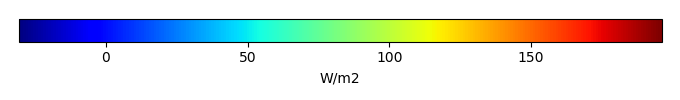MODEL MEANBIAS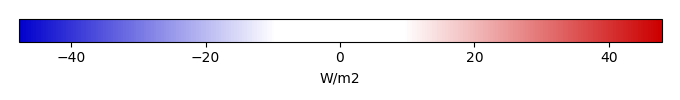BIAS SCORERMSE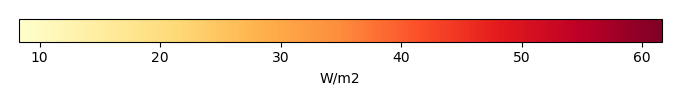RMSE SCOREBENCHMARK INTERANNUAL VARIABILITY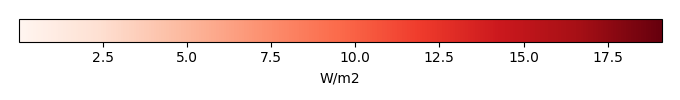MODEL INTERANNUAL VARIABILITYINTERANNUAL VARIABILITY SCOREBENCHMARK MAX MONTHMODEL MAX MONTHDIFFERENCE IN MAX MONTH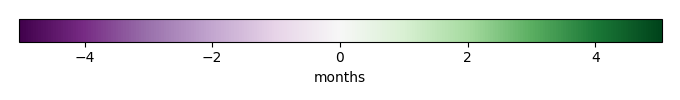SEASONAL CYCLE SCORESPATIAL TAYLOR DIAGRAMMODEL COLORS# Spatially integrated regional mean

MODEL COLORSREGIONAL MEANANNUAL CYCLEMONTHLY ANOMALYANNUAL CYCLE# All Models

BenchmarkCLM4CLM4.5CLM5# Data Information

creation_date: Thu May 8 22:58:12 PDT 2014

source_file: This product is generated from monthly 1 degree CERES EBAF Radiation observations